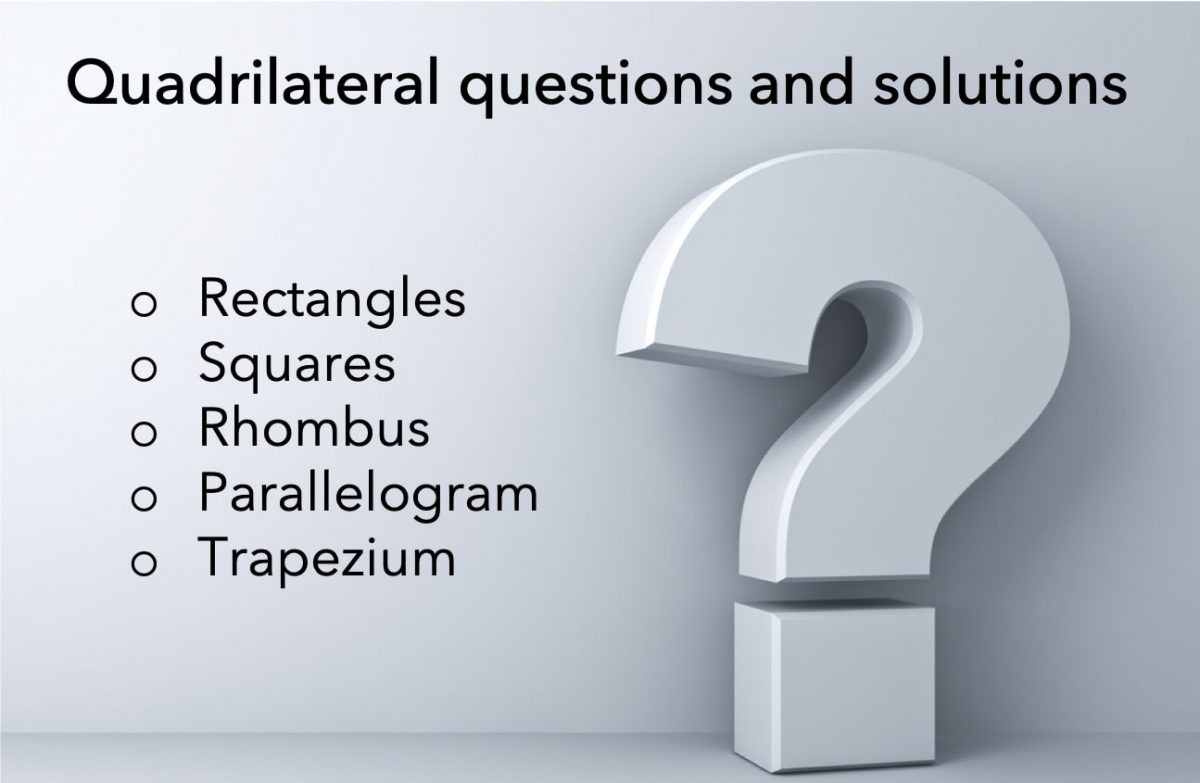# In the figure above, the vertices of ΔOPQ and ΔQRS have coordinates as indicated. Do ΔOPQ and ΔQRS have equal areas? – OG 2020 Question #325 with Solution

A 2 minute read

OG 2020: Question No. 325 In the figure above, the vertices of ΔOPQ and ΔQRS have coordinates as indicated. Do ΔOPQ and ΔQRS have equal areas? b = 2a d = 2c Source OG 2020 Type Data Sufficiency Topic Geometry Sub-Topic Triangles / Coordinate Geometry Difficulty Medium Solution Steps 1 & 2: Understand Question and

Read More# In the figure above, is the area of triangular region ADE equal to the area of rectangular region ABCD? – OGQR 2020 Question #206 with Solution

A 2 minute read

OGQR 2020: Question No. 206 In the figure above, is the area of the triangular region ADE equal to the area of rectangular region ABCD? x = 10 and y = 5. x = 2y Source OGQR 2020 Type Data Sufficiency Topic Geometry Sub-Topic Triangle/Polygons Difficulty Medium Solution Steps 1 & 2: Understand Question and Draw Inferences We are given

Read More# If rectangle ABCD is inscribed in the circle above, what is the area of the circular region? – OG 2020 Question #96 with Solution

A 2 minute read

OG 2020: Question No. 96 If rectangle ABCD is inscribed in the circle above, what is the area of the circular region? 36.00π 42.25π 64.00π 84.50π 169.00π Source OG 2020 Type Problem Solving Topic Geometry Sub-Topic Circle/Triangle Difficulty Medium Solution Given In this question, we are given Rectangle ABCD is inscribed in the circle, as

Read More# In the figure above, is the shaded region a square region? – OGQR 2020 Question #211 with Solution

A 2 minute read

OGQR 2020: Question No. 211 In the figure above, is the shaded region a square region? ABCD and EFGH are squares. BC = EF Source OGQR 2020 Type Data Sufficiency Topic Geometry Sub-Topic Polygons Difficulty Medium Solution Steps 1 & 2: Understand Question and Draw Inferences We are given: A shaded region We need to find: Whether the shaded region

Read More# On a scale drawing, a rectangle 1 inch by 1(1/3) inches represents the floor of a room and the indicated scale is 1-inch equals 15 feet. How many square tiles 6 inches on a side will be needed to cover this floor? (1 foot = 12 inches) – OG 2020 Question #100 with Solution

A < 1 minute read

OG 2020: Question No. 100 On a scale drawing, a rectangle 1 inch by 1(1/3) inches represents the floor of a room and the indicated scale is 1-inch equals 15 feet. How many square tiles 6 inches on a side will be needed to cover this floor? (1 foot = 12 inches) 40 70 120

Read More# A six-sided mosaic contains 24 triangular pieces of tile of the same size and shape, as shown in the figure above. If the sections of tile fit together perfectly, how many square centimetres of tile are in the mosaic? – OG 2020 Question #329 with Solution

A 2 minute read

OG 2020: Question No. 329 A six-sided mosaic contains 24 triangular pieces of tile of the same size and shape, as shown in the figure above. If the sections of tile fit together perfectly, how many square centimetres of tile are in the mosaic? Each side of each triangular piece of tile is 9 centimetres

Read More# Lines and Angles – Definitions, Properties, Types, Practice Questions

A 7 minute read

Ancient mathematicians introduced the concept of lines to represent straight objects which had negligible width and depth. Considered as a breadth less length by Euclid, lines form the basis of Euclidean geometry. When two rays (part of a straight line) intersect each other in the same plane, they form an angle. The point of intersection

Read More# Quadrilateral questions and solutions

A 11 minute read

Quadrilateral questions have always been a head-scratcher for a lot of candidates taking competitive or standardized exams. The primary reason behind it is learning a concept is easier as compared to applying that concept to solve questions. Therefore, to help you with the application of properties of quadrilaterals we have shared a few quadrilateral questions

Read More# Three-fourths of the area of a rectangular lawn 30 feet wide by 40 feet long is to be enclosed by a rectangular fence. If the enclosure has full width and reduced length rather than full length and reduced width, how much less fence will be needed? – OG 2020 Question #98 with Solution

A < 1 minute read

[two_third] OG 2020: Question No. 98 Three-fourths of the area of a rectangular lawn 30 feet wide by 40 feet long is to be enclosed by a rectangular fence. If the enclosure has full width and reduced length rather than full length and reduced width, how much less fence will be needed? 2(1/2) 5 10

Read More# In quadrilateral ABCD above, what is the length of AB? – OG 2020 Question #97 with Solution

A < 1 minute read

[two_third] OG 2020: Question No. 97 In quadrilateral ABCD above, what is the length of AB? √26 2√5 2√6 3√2 3√3 [/two_third] [one_third_last] Source OG 2020 Type Problem Solving Topic Geometry Sub-Topic Triangle Difficulty Medium [/one_third_last] Solution Given In this question, we are given The diagram of the quadrilateral ABCD Length of AD = 1,

Read More